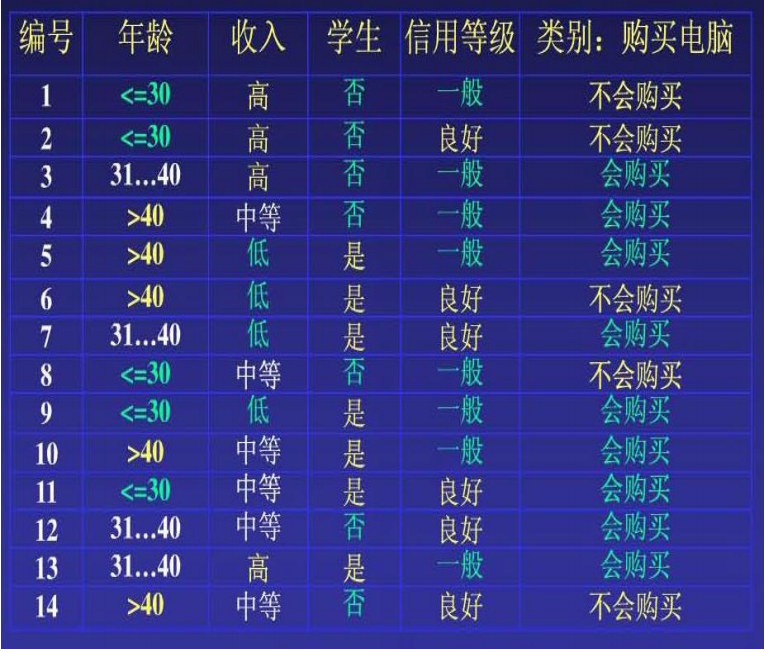## 机器学习 | 朴素贝叶斯法知识总结

zz/2023/6/4 16:15:09

## 机器学习 | 朴素贝叶斯法理论知识

(1) 设元组X=x1,x2,...,xn为一个待分类项，描述n个属性A1,A2,...An对元组的n个测量。

(2) 假定有m个类C1,C2,...,Cm。朴素贝叶斯分类法预测X属于类Ci，当且仅当P(Ci|X)≥P(Cj|X),1≤i,j≤m,i≠j 。

(3) 由于P(Ci|X)=P(X|Ci)P(Ci)P(X)，而P(X)为常数，只需P(X|Ci)P(Ci)最大即可，同时P(Ci)可求，即P(Ci)=Si/S，其中Si为样本中属于类Ci的个数，而S为样本总数，又因为特征属性是条件独立的，所以有P(X|Ci)=∏k=1nP(Xk|Ci)。

(4) 找出使P(X|Ci)P(Ci)最大的Ci，则X属于类Ci。P(C1 )=P(购买电脑=“是”) =9/14=0.643

P(C2 )= P(购买电脑=“否”) =5/14=0.357

P(年龄=“<30”|购买电脑=“是”)=2/9=0.222

P (年龄=“<30”|购买电脑=“否” )=3/5=0.6

P (收入=“中等”|购买电脑=“是” )=4/9=0.444

P (收入=“中等”|购买电脑=“否” )=2/5=0.4

P (学生=“是”|购买电脑=“是” )=6/9=0.667

P (学生=“是”|购买电脑=“否” )=1/5=0.2

P (信用率=“一般”|购买电脑=“是” )=6/9=0.667

P (信用率=“一般”|购买电脑=“否” )=2/5=0.4

P(X|购买电脑=“是”)=0.222×0.444×0.667×0.667=0.044

P(X|购买电脑=“否”)=0.6×0.4×0.2×0.4=0.019

P(C1 )=P(购买电脑=“是”) =9/14=0.643

P(C2 )= P(购买电脑=“否”) =5/14=0.357

P(X|购买电脑=“是”)×P(购买电脑=“是”)=0.028

P(X|购买电脑=“否”)×P(购买电脑=“否”)=0.007

http://www.ngui.cc/zz/2390173.html

### 手写代码(笔试面试真题)

★★★ 手写代码&#xff1a;实现forEach map filter reduce ★★★ 手写实现一个简易的 Vue Reactive ★★★ 手写代码&#xff0c;监测数组变化&#xff0c;并返回数组长度 ★★★ 手写原生继承&#xff0c;并说出局限性&#xff1f; ★★★★ 手写一个柯里化函数 ★★★…